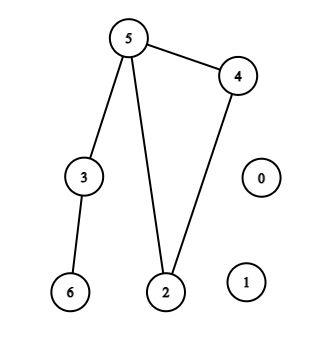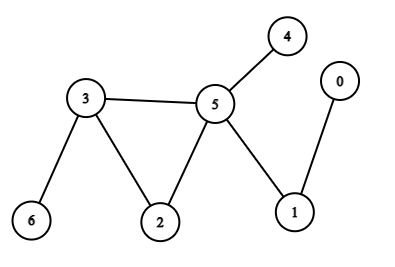Problem title
Difficulty
Avg time to solve

Maximum Sum Path Of A Binary Tree.
Hard
25 mins
Selecting Three People
Moderate
25 mins
Faulty Key
Moderate
25 mins
Sum of Big integers.
Easy
15 mins
Find the elements value if present in the cache.
Hard
35 mins
Combination Sum
Hard
45 mins
Apple Pickup
Hard
15 mins
XML encoding
Moderate
15 mins
Count Of 3s
Moderate
25 mins
Ninja and Binary Tree
Moderate
25 mins

# Find if Path Exists in Graph

Difficulty: EASY
Avg. time to solve
45 min
Success Rate
75%

Problem Statement

#### You are given an undirected graph consisting of ‘N’ nodes from 0 to ‘N’ - 1. You are given a list ‘EDGES’ of size ‘M’, consisting of all the edges of this undirected graph, and two nodes ‘SOURCE’ and ‘DESTINATION’ of this graph. Determine whether there exists a path from node ‘SOURCE’ to node ‘DESTINATION’. In other words, check whether there exists a path from node ‘SOURCE’ to node ‘DESTINATION’ by moving along the edges of the graph.

##### Note:
``````The graph has no self-edges, no parallel edges.
The graph may not be connected.
``````
##### For Example,
``````If ‘N’ = 7, ‘M’ = 5, ‘SOURCE’ = 1, ‘DESTINATION’ = 5, EDGES = [ [2, 4], [2, 5],[3, 5],[3, 6],[4, 5] ].
````````````Here, you can see that the graph is not connected and there is no way we can reach node 5 from node 1 as they both are in different connected components. Hence, the output is “No”.
``````
##### Input Format :
``````The first line of input contains an integer ‘T’ denoting the number of test cases. then ‘T’ test cases follow.

The first line of each test case consists of four space-separated integers  ‘N’, ‘M’, ‘SOURCE’, ‘DESTINATION’, described in the problem statement.

Then next ‘M’ lines follow in each test case. The ith line consists of two space-separated integers ‘EDGES[i]’ and ‘EDGES[i]’ representing that there is a undirected edge between nodes ‘EDGES[i]’ and ‘EDGES[i]’.
``````
##### Output Format :
``````For each test case, print the “Yes” if there exists a path from node ‘SOURCE’ to node ‘DESTINATION’ by moving along the edges of the graph, Otherwise, print “No”.

Print a separate line for each test case.
``````
##### Note :
``````You do not need to print anything, it has already been taken care of. Just implement the given function.
``````
##### Constraints:
``````1 <= T <= 10
1 <= N <= 500
1 <= M <= (N*(N-1)) / 2
0 <= ‘SOURCE’, ‘DESTINATION’ <= N - 1
Time limit: 1 sec
``````
##### Sample Input 1 :
``````2
7 5 1 5
2 5
2 4
3 5
3 6
4 5
7 7 1 6
0 1
1 5
2 5
2 3
5 3
5 4
6 3
``````
##### Sample output 1 :
`````` No
Yes
``````
##### Explanation For Sample Output 1:
``````For the first test case, the graph will be:
````````````Here, you can see that the graph is not connected and there is no way such that we can reach node 5 from node 1 as they both are in different connected components. Hence, the output is “No”.

For the second test case, the graph will be:
````````````Here, source = 1 , destination = 6, We can reach node 6 from node 1 as 1 -> 5 -> 3 ->6.
Hence, the output is “Yes”.
``````
##### Sample Input 2 :
``````2
6 8 5 1
0 4
0 5
1 2
1 5
3 5
3 4
4 2
5 2
10 14 4 8
0 2
1 8
1 0
2 6
3 1
3 7
4 7
5 7
5 6
6 7
8 2
8 0
9 2
9 6
``````
##### Sample output 2 :
``````Yes
Yes
``````Console